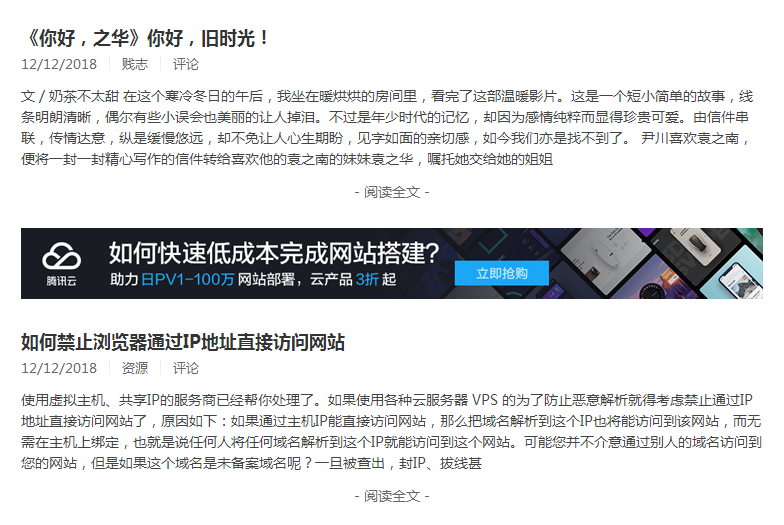### Typecho在文章列表中插入广告的方法

<?php while($this->next()): ?> <?php$counter++; ?>
<?php if($counter==4) :?> 这里填写广告代码 <?php else: ?> <?php endif; ?> 这是通过if语句判断来实现的，<?php if($counter==4) :?>中的数字4代表广告出现的位置，4就是广告出现在第3篇文章摘要的下面。

{template:post-istop}
{else}
{template:post-multi}
{/if}
{/foreach}

{php}$j=1;{/php} {foreach$articles as $article} {if$article.IsTop}
{template:post-istop}
{if $j==3} 你的广告代码 {/if} {php}$j++;{/php}
{else}
{template:post-multi}
{/if}
{/foreach}

{php}$i=1;{/php} {foreach$articles as $article} {if$article.IsTop}
{template:post-istop}
{else}
{template:post-multi}
{if $i==3} 你的广告代码 {/if} {php}$i++;{/php}
{/if}
{/foreach}

{php}$i=1;$j=1;{/php}
{foreach $articles as$article}
{if $article.IsTop} {template:post-istop} {if$j==3}
你的广告代码
{/if}
{php}$j++;{/php} {else} {template:post-multi} {if$i==3}
{/foreach}